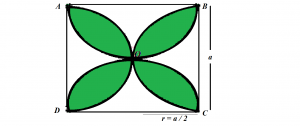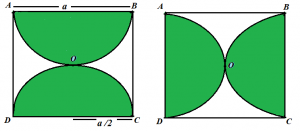Related Articles
Find the area of the shaded region formed by the intersection of four semicircles in a square
• Difficulty Level : Medium
• Last Updated : 04 Apr, 2019

Given the length of the side of a square a, the task is to find the area of the shaded region formed by the intersection of four semicircles in a square as shown in the image below:Examples:

Input: a = 10
Output: 57

Input: a = 19
Output: 205.77

## Recommended: Please try your approach on {IDE} first, before moving on to the solution.

Approach: Area of the shaded region will be:

Area(semicircle1) + Area(semicircle2) + Area(semicircle3) + Area(semicircle4) – Area(square).

Since all semicircles are of same radius, therefore, area of all semicircles will be equal. So, the above formula can be written as:
4*(Area of Semicircle) – Area(Square)

The area of a semicircle is (3.14 * r2) / 2 where r is the radius of the semicircle which is equal to a / 2.

Hence, Area of the shaded region = 4 * (3.14 * (a * a) / 8 ) – a * aBelow is the implementation of the above approach:

## C++

 `// C++ implementation of the approach ` `#include ` `using` `namespace` `std; ` ` `  `// Function to return the area ` `// of the shaded region ` `float` `findAreaShaded(``float` `a) ` `{ ` ` `  `    ``// Area of the square ` `    ``float` `sqArea = a * a; ` ` `  `    ``// Area of the semicircle ` `    ``float` `semiCircleArea = (3.14 * (a * a) / 8); ` ` `  `    ``// There are 4 semicircles ` `    ``// shadedArea = Area of 4 semicircles - Area of square ` `    ``float` `ShadedArea = 4 * semiCircleArea - sqArea; ` ` `  `    ``return` `ShadedArea; ` `} ` ` `  `// Driver code ` `int` `main() ` `{ ` `    ``float` `a = 10; ` `    ``cout << findAreaShaded(a); ` ` `  `    ``return` `0; ` `} `

## Java

 `// Java implementation of the approach ` `class` `GFG { ` ` `  `    ``// Function to return the area ` `    ``// of the shaded region ` `    ``static` `float` `findAreaShaded(``float` `a) ` `    ``{ ` ` `  `        ``// Area of the square ` `        ``float` `sqArea = a * a; ` ` `  `        ``// Area of the semicircle ` `        ``float` `semiCircleArea = (``float``)(``3.14` `* (a * a) / ``8``); ` ` `  `        ``// There are 4 semicircles ` `        ``// shadedArea = Area of 4 semicircles - Area of square ` `        ``float` `ShadedArea = ``4` `* semiCircleArea - sqArea; ` ` `  `        ``return` `ShadedArea; ` `    ``} ` ` `  `    ``// Driver code ` `    ``public` `static` `void` `main(String[] args) ` `    ``{ ` `        ``float` `a = ``10``; ` `        ``System.out.println(findAreaShaded(a)); ` `    ``} ` `} `

## Python3

 `# Python3 implementation of the approach ` ` `  `# Function to return the area  ` `# of the shaded region ` `def` `findAreaShaded(a): ` `     `  `    ``# Area of the square ` `    ``sqArea ``=` `a ``*` `a; ` ` `  `    ``# Area of the semicircle ` `    ``semiCircleArea ``=` `(``3.14` `*` `(a ``*` `a ) ``/` `8``) ` ` `  `    ``# There are 4 semicircles ` `    ``# shadedArea = Area of 4 semicircles - Area of square ` `    ``ShadedArea ``=` `4` `*` `semiCircleArea ``-` `sqArea ;  ` ` `  `    ``return` `ShadedArea; ` ` `  `# Driver code ` `if` `__name__ ``=``=` `'__main__'``: ` `    ``a ``=` `10` `    ``print``(findAreaShaded(a)) `

## C#

 `// C# implementation of the approach ` `using` `System; ` ` `  `class` `GFG  ` `{ ` ` `  `    ``// Function to return the area ` `    ``// of the shaded region ` `    ``static` `float` `findAreaShaded(``float` `a) ` `    ``{ ` ` `  `        ``// Area of the square ` `        ``float` `sqArea = a * a; ` ` `  `        ``// Area of the semicircle ` `        ``float` `semiCircleArea = (``float``)(3.14 * (a * a) / 8); ` ` `  `        ``// There are 4 semicircles ` `        ``// shadedArea = Area of 4 semicircles - Area of square ` `        ``float` `ShadedArea = 4 * semiCircleArea - sqArea; ` ` `  `        ``return` `ShadedArea; ` `    ``} ` ` `  `    ``// Driver code ` `    ``public` `static` `void` `Main() ` `    ``{ ` `        ``float` `a = 10; ` `        ``Console.WriteLine(findAreaShaded(a)); ` `    ``} ` `} ` ` `  `// This code is contributed by mohit kumar 29 `

## PHP

 ` `

Output:

```57
```

Attention reader! Don’t stop learning now. Get hold of all the important DSA concepts with the DSA Self Paced Course at a student-friendly price and become industry ready.

My Personal Notes arrow_drop_up
Recommended Articles
Page :